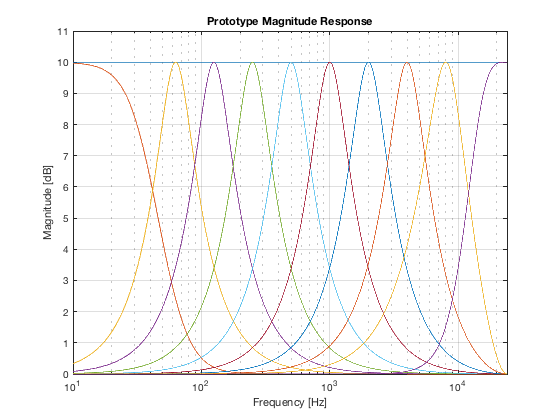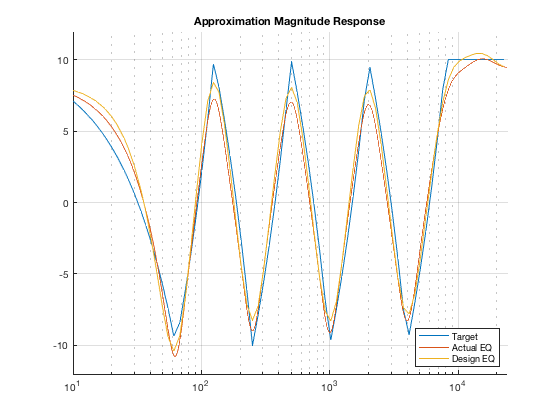## All About Audio Equalization: Solutions and Frontiers

by V. Välimäki and J. Reiss, in Applied Sciences, vol. 6, no. 5, p. 129, May 2016.

Reproduce in Code (c) Sebastian Jiro Schlecht: Monday, 7. January 2019

```close all; clear; clc;
```

## Initialization

Setup variables such as sampling frequency, center frequencies and control frequencies, and command gains

```fs = 48000;
fftLen = 2^16;

centerFrequencies = [ 63, 125, 250, 500, 1000, 2000, 4000, 8000]; % Hz
ShelvingCrossover = [46 11360]; % Hz
numFreq = length(centerFrequencies) + length(ShelvingCrossover);
R = 2.7;

% control frequencies are spaced logarithmically
numControl = 100;
controlFrequencies = round(logspace(log10(1), log10(fs/2.1),numControl+1));

% target magnitude response via command gains
targetF = [1, centerFrequencies fs];
targetG = [1; -1; 1; -1; 1; -1; 1; -1; 1; 1]*10; % dB
targetInterp = interp1(targetF, targetG, controlFrequencies)';
```

## desgin prototype of the biquad sections

```prototypeGain = 10; % dB
prototypeGainArray = prototypeGain * ones(numFreq+1,1);
prototypeSOS = proportionalParametricEQ(centerOmega, shelvingOmega, R, prototypeGainArray);
[G,prototypeH,prototypeW] = probeSOS (prototypeSOS, controlFrequencies, fftLen, fs);
G = G / prototypeGain; % dB vs control frequencies

% plot
figure(1);
semilogx(prototypeW,mag2db(abs(prototypeH)))
ylim([0 11])
xlim([10 fs/2])
grid on;
title('Prototype Magnitude Response')
xlabel('Frequency [Hz]')
ylabel('Magnitude [dB]')
```## compute optimal parametric EQ gains

Either you can use a unconstrained linear solver or introduce gain bounds at [-20dB,+20dB] with acceptable deviation from the self-similarity property. The plot shows the deviation between design curve and actual curve.

```upperBound = [Inf, 2 * prototypeGain * ones(1,numFreq)];
lowerBound = -upperBound;

optG = lsqlin(G, targetInterp, [],[],[],[], lowerBound, upperBound);
% optG = G\targetInterp; % unconstrained solution
optimalSOS = proportionalParametricEQ( centerOmega, shelvingOmega, R, optG );

% plot
figure(2); hold on; grid on;

[hOpt,wOpt] = freqz(optimalSOS,fftLen,fs);
plot(controlFrequencies, targetInterp);
plot(wOpt,mag2db(abs(hOpt)))
plot(controlFrequencies, G*optG)

set(gca, 'xScale', 'log')
ylim([-12 12])
xlim([10 fs/2])
title('Approximation Magnitude Response')
legend('Target', 'Actual EQ', 'Design EQ','Location','SouthEast');
```
```Minimum found that satisfies the constraints.

Optimization completed because the objective function is non-decreasing in
feasible directions, to within the default value of the optimality tolerance,
and constraints are satisfied to within the default value of the constraint tolerance.

``````function rad = hertz2rad(hertz, fs)
% Author: Sebastian J Schlecht
% Date: 24.03.2017

rad = hertz / fs * 2*pi;
```
probeSOS
```function [G,H,W] = probeSOS (SOS, controlFrequencies, fftLen, fs)
% Probe the frequency / magnitude response of a cascaded SOS filter at the points
% specified by the control frequencies
%
% Author: Sebastian J Schlecht
% Date: 24.03.2017

numFreq = size(SOS, 1);

H = zeros(fftLen, numFreq);
W = zeros(fftLen, numFreq);
G = zeros(length(controlFrequencies), numFreq);

for band = 1:numFreq

b = SOS(band, 1:3);
a = SOS(band, 4:6);

[h,w] = freqz(b,a,fftLen,fs);

g = interp1(w,mag2db(abs(h)),controlFrequencies);

G(:,band) = g;
H(:,band) = h;
W(:,band) = w;
end
```
proportionalParametricEQ
```function SOS = proportionalParametricEQ( centerOmega, shelvingOmega, R, gaindB )
% Proportional parametric graphical EQ as described in
% 	V. Välimäki and J. Reiss, "All About Audio Equalization: Solutions and Frontiers,"
%       Applied Sciences, vol. 6, no. 5, p. 129, May 2016.
% 	J. M. Jot, "Proportional Parametric Equalizers - Application to Digital
%       Reverberation and Environmental Audio Processing," presented at the
%       Proc. Audio Eng. Soc. Conv., New York, NY, USA, 2015, pp. 1-8.
%
% The frequency bands are described by the centerOmega and shelvingOmega
% R relates to the quality factor and
% gaindB is the command gain of the bands
%
% Author: Sebastian J Schlecht
% Date: 24.03.2017

numFreq = length(centerOmega) + length(shelvingOmega) + 1;
assert( length(gaindB) == numFreq);
SOS = zeros(numFreq,6);

for band = 1:numFreq
switch band
case 1
b = [1 0 0] * db2mag(gaindB(band));
a = [1 0 0];
case 2
[b,a] = shelvingFilter( shelvingOmega(1), db2mag(gaindB(band)), 'low' );
case numFreq
[b,a] = shelvingFilter( shelvingOmega(2), db2mag(gaindB(band)), 'high' );
otherwise
Q = sqrt(R) / (R-1);
[b,a] = bandpassFilter( centerOmega(band-2), db2mag(gaindB(band)), Q );
end

sos = [b a];

SOS(band,:) = sos;
end
```